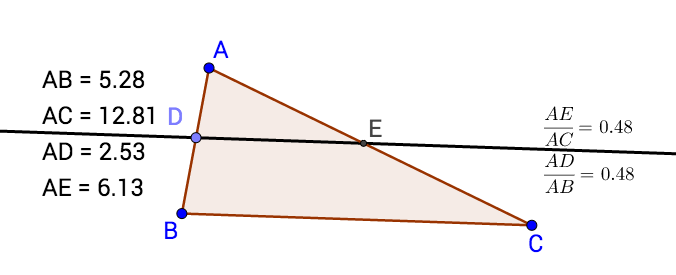# Geo-2017-Constructions-Triangle Inequalities & Similarities

## Objective

Demonstrate the Hinge Theorem by isosceles triangles.

## Hinge Theorem

1. Construct two isosceles triangle using the center of a circle and two points on its circumference.
2. Measure the central angle﻿and sides﻿opposite them.
3. Compare the lengths of the sides and create a conjecture between the lengths of the side

## Hinge Theorem Conjecture

Compare the measure of the central angles and the sides of the triangles opposite them.

## Similar Triangles

﻿1. Construct a triangle with a line parallel to one of the sides.
2. Measure AB, AC, AD and AE.

## Similar Triangle: Proportional Sides

Calculate the following ratios: How do those those ratio change or not as you drag the vertices of the triangle?

## AA Proof of Similar Triangles

Any two triangles that have two congruent angles always have proportional sides. Prove that the two triangles ABC and ADE are similar by showing that they have two congruent corresponding angles.